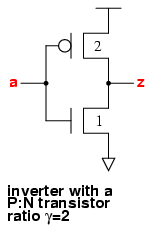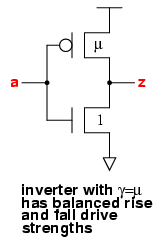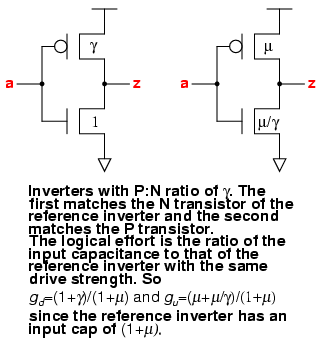## Quick Review of Logical Effort

UP PREV NEXT

The book Logical Effort by Ivan Sutherland, Bob Sproull and David Harris presents the basic theory and equations for logical effort. The logical effort of a gate is a way of expressing how difficult it is for that gate to drive its output, and how that relates to its delay.

We are interested here in designing a standard cell library which contains cells that are fast, and so their delay is important to us. We give a priority to including cells with a low logical effort since these will be faster, and we take care to choose a suitable P:N transistor ratio.

### Logical Effort of Inverters with Different P:N Transistor RatiosThe Logical Effort book considers the logical effort of different gates. An inverter with a P:N transistor ratio (called gamma, γ) of 2 is defined to have a logical effort of 1, and other gates are referenced to this.Inverters with different values of gamma will have different logical efforts. The inverter which should be used as the reference inverter is the one with balanced rise and fall drive strengths. We define the conductivity ratio between N and P transistors as µ, where the conductivity of N transistors is µ× the conductivity of P transistors. The value of µ varies between processes, but for the vsclib a value of µ=2.25 has been used. This means that an inverter where the P transistor is 2.25× bigger than the N transistor has balanced rise and fall drive strengths and is used as our reference inverter for calculating logical effort.

In Logical Effort, a value of µ=2 is normally used, so that in the examples γ=µ, which is a particular case. We are interested here in seeing the effect of using inverters and other gates where γ≠µ.

Using a value of γ=2 is common practice, and has been chosen for the vsclib, even if the conductivity of the P transistors is less than half that of the N ones. It has the advantage of being simple, and as we shall see, the fastest gates generally have a P:N transistor ratio less than µ.The conductivity ratio, µ, depends upon the process and normally lies between 2 and 3. For the vsclib the value of µ=2.25 has been chosen for convenience, since the factor √µ is often used. If µ =2.25, √µ=1.5, which makes the numbers easier.

The logical effort of a gate is denoted by g, and is the average of the rising logical effort gu and the falling logical effort gd.
g = ½(gd+gu)
The falling logical effort of a gate is determined by setting the conductivity of the N transistor to be equal to the reference inverter's, scaling the P transistor size, and using the ratio of the input capacitance. For an inverter with a P:N ratio of γ, we set the N transistor size = 1, the P transistor size = γ, and the falling logical effort is then:
gd = (1+γ)/(1+µ)
For the rising logical effort, the P transistor size is set equal to µ which means that the N transistor must be scaled down to µ/γ. This gives a rising logical effort of:
gu = ( µ+µ/γ)/(1+µ)
The logical effort g is then:
g = ½×(γ+1+µ+ µ/γ)/(1+µ)

Some values of logical effort for inverters with different values of γ, assuming that µ=2.25, are:

 P:N ratio γ 1 1.5 2 2.25 2.5 3 4 logical effort g 1 25/26 51/52 1 133/130 14/13 125/104 1.00 0.96 0.98 1.00 1.02 1.08 1.20

In the vsclib, γ = 2 has been chosen for the inverters because this represents a good compromise between speed and balanced rise and fall drive strengths, and also because it is simple and follows widely used industry practice.

The values of logical effort are different to the ones used in the Logical Effort book. In most of the examples, the logical effort of an inverter is simply given as 1, even in the case where γ and µ are different. For example, in the case of an inverter with γ=2 and µ=3 (Table 7.1),
gd = 4/5; gu = 6/5; and g = 1.
However, using the formulae above,
gd = 3/4; gu = 9/8; and g = 15/16. The difference comes from normalising the result to 1 by scaling by 16/15. In fact, 3/4×16/15=4/5 and 9/8×16/15=6/5. There doesn't seem to be any reason to normalise the logical effort so that an inverter with γ=2 has a logical effort of 1, regardless of the value being used for the mobility µ. Setting the logical effort of an inverter with &gamma=2 to 1 keeps it simple, but in this case it is better to set µ=2 as well. Once µ≠1, then it is better to use the correct values for inverter logical effort.

### P:N Transistor Ratio Giving Fastest Inverter

The logical effort g varies as γ varies, and we can find the minimum value of g by differentiating with respect to γ and setting the differential to zero.
g = ½×(γ+1+µ+ µ/γ)/(1+µ)
dg/dγ = 0 = 1-µ2
from which γ = √µ for the fastest inverter. If µ=2.25, then the fastest inverter has γ=1.5, which can be seen in the table above.